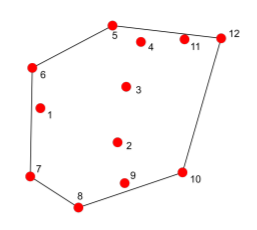# Does anyone know why it is also taking point 9 as convex hull point eventhough it shouldn't?

1 view (last 30 days)
The Merchant on 4 Dec 2019
Commented: Rena Berman on 16 Jan 2020
Does anyone know why it is also taking point 9 as convex hull point eventhough it shouldn't?
Image representing the cloud of points and it's convex hullInput
clear;
close all;
clc;
xy = [
3 12 % Point 1
10 8 % Point 2
11 14 % Point 3
13 16 % Point 4
9 19 % Point 5
1 15 % Point 6
2 5 % Point 7
6 1 % Point 8
12 4 % Point 9
16 6 % Point 10
14 17 % Point 11
19 18 % Point 12
];
xy = xy';
convexHull = getConvexHull(xy);
function k = getConvexHull(xy)
[m,n] = size(xy);
if m~=2
error('convexhull: xy must have 2 columns');
end
[xmin,first] = min( xy(1,:) );
ind = [1:(first-1) (first+1):n];
angle = atan2( xy(1,ind)-xy(1,first), xy(2,ind)-xy(2,first) );
[junk,order] = sort(angle);
ind = [ind(order) first];
stack = zeros( n, 1, 'uint32' );
stack(1) = first;
stacktop = 1;
i = 1;
while i<=n
if stacktop<2
stacktop = stacktop+1;
stack(stacktop) = ind(i);
i = i+1;
else
p0 = xy(:,stack(stacktop));
p1 = xy(:,stack(stacktop-1));
p2 = xy(:,ind(i));
if (p1(1)-p0(1))*(p2(2)-p0(2))-(p2(1)-p0(1))*(p1(2)-p0(2)) >= 0
stacktop = stacktop+1;
stack(stacktop) = ind(i);
i = i+1;
else
stacktop = stacktop-1;
end
end
end
k = stack(1:stacktop);
end
Output
6
5
12
10
9
8
7
6
(Counter clockwise convex hull points)
Rena Berman on 16 Jan 2020
(Answers Dev) Restored edit

Philippe Lebel on 4 Dec 2019
Edited: Philippe Lebel on 4 Dec 2019
By observation, points 8, 9 and 10 are on the same line.
You have to add a condition to decimate redundant points. (points that lie on the same line or points that are stacked on each other for example)
You can quickly verify if what i say is the case by changing point 9 by [4.00001,12]
##### 2 CommentsShowHide 1 older comment
Philippe Lebel on 4 Dec 2019
I just saw your picture, i don't know why point 9 shows inside the convex hull. Maybe it has been made with an other data set?